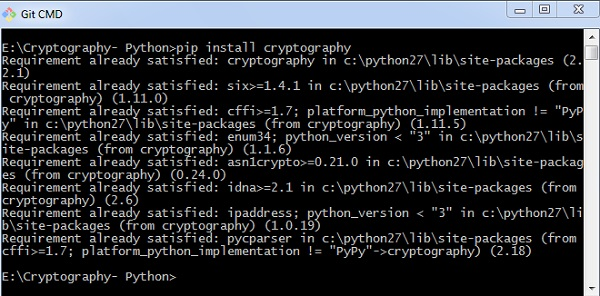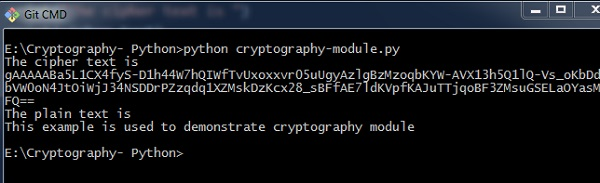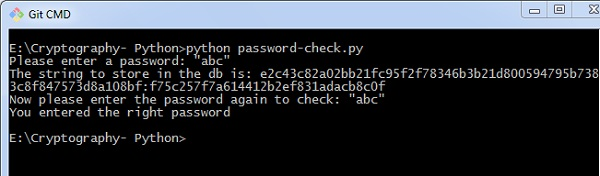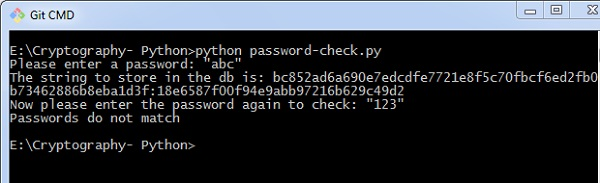# Python Modules of Cryptography

In this chapter, you will learn in detail about various modules of cryptography in Python.

## Cryptography Module

It includes all the recipes and primitives, and provides a high level interface of coding in Python. You can install cryptography module using the following command −

```>pip install cryptography
```### Code

You can use the following code to implement the cryptography module −

```from cryptography.fernet import Fernet
key = Fernet.generate_key()
cipher_suite = Fernet(key)
cipher_text = cipher_suite.encrypt("This example is used to demonstrate cryptography module")
plain_text = cipher_suite.decrypt(cipher_text)```

### Output

The code given above produces the following output −The code given here is used to verify the password and creating its hash. It also includes logic for verifying the password for authentication purpose.

```import uuid
import hashlib

# uuid is used to generate a random number of the specified password
salt = uuid.uuid4().hex
return hashlib.sha256(salt.encode() + password.encode()).hexdigest() + ':' + salt

print('The string to store in the db is: ' + hashed_password)

else:

### Output

Scenario 1 − If you have entered a correct password, you can find the following output −Scenario 2 − If we enter wrong password, you can find the following output −### Explanation

Hashlib package is used for storing passwords in a database. In this program, salt is used which adds a random sequence to the password string before implementing the hash function.